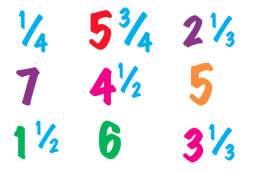# Product minus sum

How much is the product of 6 1/2 and 4 1/3 more than the sum of 3 1/3 and 2 1/6?

x =  22 23 = 68/3

### Step-by-step explanation:Did you find an error or inaccuracy? Feel free to write us. Thank you!

Tips for related online calculators
Need help calculating sum, simplifying, or multiplying fractions? Try our fraction calculator.
Need help with mixed numbers? Try our mixed-number calculator.